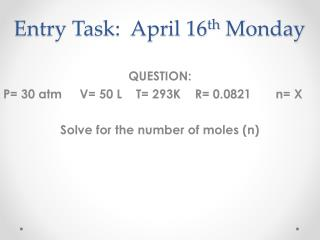Download PresentationEntry Task: April 16 th Monday

# Entry Task: April 16 th Monday - PowerPoint PPT PresentationDownload Presentation## Entry Task: April 16 th Monday

- - - - - - - - - - - - - - - - - - - - - - - - - - - E N D - - - - - - - - - - - - - - - - - - - - - - - - - - -
##### Presentation Transcript

1. Entry Task: April 16th Monday QUESTION: P= 30 atm V= 50 L T= 293K R= 0.0821 n= X Solve for the number of moles (n)

2. Agenda • Go over Combined and Ideal ws • HW: Pre-Lab Proving gas law

3. Provide the equation for the combined gas law. P1 V1 = P2 V2 T1 T2

4. 1. If a gas occupies a volume of 100 cm3 at a pressure of 101.3 kPa and 27C, what volume will the gas occupy at 120 kPa and 50C? P1 = V1= T1= 100cm3 27 + 273 = 300K 101.3 kPa 50 + 273 = 323K X cm3 P2 = V2 = T2 = 120 kPa

5. P1 = V1= T1= 100cm3 27 + 273 = 300K 101.3 kPa 50 + 273 = 323K X cm3 P2 = V2 = T2 = 120 kPa (101.3 kPa)(100cm3) = (120 kPa) (X cm3) 323 K 300 K

6. GET X by its self!! (101.3 kPa)(100cm3) = (120 kPa) (X cm3) 323 K 300 K (101.3 kPa)(100 cm3)(323K) = X cm3 (300 K)(120 kPa)

7. DO the MATH (101.3)(100 cm3)(323) = X cm3 (300 )(120) 3271990 cm3 = 90.9 cm3 36000

8. 2. A toy balloon has an internal pressure of 1.05 atm and a volume of 5.0 L. If the temperature where the balloon is released is 20˚ C, what will happen to the volume when the balloon rises to an altitude where the pressure is 0.65 atm and the temperature is –15˚ C? P1 = V1= T1= 20 + 273 = 293K 5.0 L 1.05 atm P2 = V2 = T2 = 0.65 atm -15 + 273 = 258K X L

9. P1 = V1= T1= 20 + 273 = 293K 5.0 L 1.05 atm P2 = V2 = T2 = 0.65 atm -15 + 273 = 258K X L (1.05 atm) (5.0L) = (0.65 atm) (XL) 258 K 293 K

10. GET X by its self!! (1.05 atm) (5.0L) = (0.65 atm) (XL) 258 K 293 K (1.05 atm)(5.0L)(258K) = X L (293 K)(0.65 atm)

11. DO the MATH (1.05)(5.0L)(258) = X L (293)(0.65) 1355 = 7.11L 190.5

12. 3. A closed gas system initially has volume and temperature of 2.7 L and 466 K with the pressure unknown. If the same closed system has values of 1.01 atm, 4.70 L and 605 K, what was the initial pressure in atm? P1 = V1= T1= 466K 2.7L X atm 4.70 L P2 = V2 = T2 = 1.01 atm 605K

13. P1 = V1= T1= 466K 2.7L X atm 4.70 L P2 = V2 = T2 = 1.01 atm 605K (X atm) (2.7L) = (1.01atm) (4.70L) 605 K 466 K

14. GET X by its self!! (X atm) (2.7L) = (1.01atm) (4.70L) 605 K 466 K (466K)(1.01 atm)(4.70L) = X atm (2.7L)(605 K)

15. DO the MATH (466)(1.01 atm)(4.70) = X atm (2.7)(605 ) 2212 = 1.35 atm 1634

16. 4. A closed gas system initially has pressure and temperature of 153.3 kPa and 692.0°C with the volume unknown. If the same closed system has values of 32.26 kPa, 7.37 L and -48.00°C, what was the initial volume in L? P1 = V1= T1= 692.0+ 273= 965K X L 153.3 kPa 7.37 L P2 = V2 = T2 = 32.26 kPa -48.0 + 273= 225K

17. P1 = V1= T1= 692.0+ 273= 965K X L 153.3 kPa 7.37 L P2 = V2 = T2 = 32.26 kPa -48.0 + 273= 225K (153.3 kPa)(XL) = (32.26 kPa) (7.37L) 225 K 965 K

18. GET X by its self!! (153.3 kPa)(XL) = (32.26 kPa) (7.37L) 225 K 965 K (965K)(32.26kPa)(7.37L) = X L (153.3 kPa)(225 K)

19. DO the MATH (965)(32.26)(7.37L) = X L (153.3)(225) 229435 = 6.65 L 34493

20. 1.00 atm X 100L 0.0821 4.0 mol (4.0 mol)(0.0821)(X) (1.00 atm)(100L) = 5. At what temperature (in Kelvin) would 4.0 moles of Hydrogen gas in a 100 liter container exert a pressure of 1.00 atmospheres? P= V= T= R= n=

21. Get X by itself! (4.0 mol)(0.0821)(X) (1.00 atm)(100L) = X (1.00 atm)(100L) = (4.0) (0.0821) 100 = 305 K 0.3284

22. 0.5 mol 18 L 45+273= 318K X atm 0.0821 (0.5 mol)(0.0821)(318K) (X atm)(18 L) = 6. An 18 liter container holds 16.00 grams of O2 at 45°C. What is the pressure (atm) of the container? P= V= T= R= n=

23. Get X by itself! (0.5 mol)(0.0821)(318K) (X atm)(18 L) = X (0.5)(0.0821)(318) = (18) 13.1 = 0.73 atm 18

24. 3.00 L 2.00 atm 0.0821 X mol 25+273= 298 K (X mol)(0.0821)(298K) (2.00 atm)(3.00L) = 7. How many moles of oxygen must be in a 3.00 liter container in order to exert a pressure of 2.00 atmospheres at 25 °C? P= V= T= R= n=

25. Get X by itself! (X mol)(0.0821)(298K) (2.00 atm)(3.00L) = (2.00)(3.00) = X (0.0821)(298) 6.00 = 0.245 mol 24.47

26. 26+273=299K 2.3 atm 0.0026L 0.0821 X mol (X mol)(0.0821)(299) (2.3 atm)(0.0026L) = 8. A flashbulb of volume 0.0026 Lcontains O2 gas at a pressure of 2.3 atm and a temperature of 26C. How many moles of O2 does the flashbulb contain? P= V= T= R= n=

27. Get X by itself (X mol)(0.0821)(299) (2.3 atm)(0.0026L) = (2.3)(0.0026) = X (0.0821)(299) 0.00598 24.55 = 0.00024 mol of O2 OR 2.4 x 10-4mol

28. Homework: Pre-LabProving “R” constant